##### The Japanese Bonsai specialist
Direct order Contact Help / Services Newsletter# Set of sphagnum and 5 air layering baby rooter pot

Marcottage aérien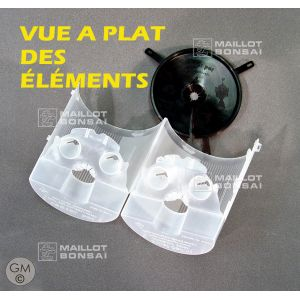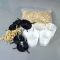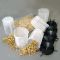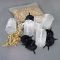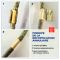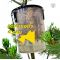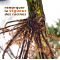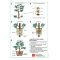ref. : 482

17,80

Available quantity : 9Order

###### Description

Set of 5 pieces with one bag of sphagnum peat moss. Height 100 mm, Ø 80 mm. For trunks of 7-15 mm diameter.

Plastic rooter pot with a convenient side opening and water reservoir. This pot is for propagating all trees and bushes using a system called air layering. Use it to form bonsai or simply to clone plants that are difficult to sow from seed or grow from cuttings.

Choose a branch on a mother plant, strip off a piece of bark and place the rooter pot around the wound, filled with sphagnum or a mix of sphagnum and Japanese akadama earth. Close the pot using the hooks and put on the lid to encourage rainwater and moisture to collect through its funnel-shaped top. A water reserve at the bottom of the pot ensures sufficient hydration for the soil. This handy pot is reusable and economic. The time it takes for roots to develop will vary from 1 to 3 months depending on the species. Once small roots appear, cut the branch with secateurs just under the pot to remove the newly rooted plant from the mother plant. Now you can take away the rooter pot and transplant your new plant into a container or directly into the soil, taking care not to break the fragile roots.

#sphagnum 5.8 #rooter 5.6 #plant 4.5 #with 4.4 #from 4.4 #layering 3.8 #bonsai 3.6 #roots 3.5 #branch 2.6 #mother 2.6

Formule
(( ROUND((CHAR_LENGTH(b.article_nom)-CHAR_LENGTH(REPLACE(b.article_nom, 'sphagnum', '')))/LENGTH('sphagnum')) + ROUND((CHAR_LENGTH(b.article_description)-CHAR_LENGTH(REPLACE(b.article_description, 'sphagnum', '')))/LENGTH('sphagnum')) ) * 5.8) + (( ROUND((CHAR_LENGTH(b.article_nom)-CHAR_LENGTH(REPLACE(b.article_nom, 'rooter', '')))/LENGTH('rooter')) + ROUND((CHAR_LENGTH(b.article_description)-CHAR_LENGTH(REPLACE(b.article_description, 'rooter', '')))/LENGTH('rooter')) ) * 5.6) + (( ROUND((CHAR_LENGTH(b.article_nom)-CHAR_LENGTH(REPLACE(b.article_nom, 'plant', '')))/LENGTH('plant')) + ROUND((CHAR_LENGTH(b.article_description)-CHAR_LENGTH(REPLACE(b.article_description, 'plant', '')))/LENGTH('plant')) ) * 4.5) + (( ROUND((CHAR_LENGTH(b.article_nom)-CHAR_LENGTH(REPLACE(b.article_nom, 'with', '')))/LENGTH('with')) + ROUND((CHAR_LENGTH(b.article_description)-CHAR_LENGTH(REPLACE(b.article_description, 'with', '')))/LENGTH('with')) ) * 4.4) + (( ROUND((CHAR_LENGTH(b.article_nom)-CHAR_LENGTH(REPLACE(b.article_nom, 'from', '')))/LENGTH('from')) + ROUND((CHAR_LENGTH(b.article_description)-CHAR_LENGTH(REPLACE(b.article_description, 'from', '')))/LENGTH('from')) ) * 4.4) + (( ROUND((CHAR_LENGTH(b.article_nom)-CHAR_LENGTH(REPLACE(b.article_nom, 'layering', '')))/LENGTH('layering')) + ROUND((CHAR_LENGTH(b.article_description)-CHAR_LENGTH(REPLACE(b.article_description, 'layering', '')))/LENGTH('layering')) ) * 3.8) + (( ROUND((CHAR_LENGTH(b.article_nom)-CHAR_LENGTH(REPLACE(b.article_nom, 'roots', '')))/LENGTH('roots')) + ROUND((CHAR_LENGTH(b.article_description)-CHAR_LENGTH(REPLACE(b.article_description, 'roots', '')))/LENGTH('roots')) ) * 3.5) + (( ROUND((CHAR_LENGTH(b.article_nom)-CHAR_LENGTH(REPLACE(b.article_nom, 'mother', '')))/LENGTH('mother')) + ROUND((CHAR_LENGTH(b.article_description)-CHAR_LENGTH(REPLACE(b.article_description, 'mother', '')))/LENGTH('mother')) ) * 2.6) + (( ROUND((CHAR_LENGTH(b.article_nom)-CHAR_LENGTH(REPLACE(b.article_nom, 'branch', '')))/LENGTH('branch')) + ROUND((CHAR_LENGTH(b.article_description)-CHAR_LENGTH(REPLACE(b.article_description, 'branch', '')))/LENGTH('branch')) ) * 2.6) + (( ROUND((CHAR_LENGTH(b.article_nom)-CHAR_LENGTH(REPLACE(b.article_nom, 'using', '')))/LENGTH('using')) + ROUND((CHAR_LENGTH(b.article_description)-CHAR_LENGTH(REPLACE(b.article_description, 'using', '')))/LENGTH('using')) ) * 2.5)

## Secure payment## Delivery

Our logistic partners :04 74 55 23 48
Pépinière MAILLOT-BONSAÏ
Le Bois Frazy
01990 RELEVANT - FRANCE
on appointment